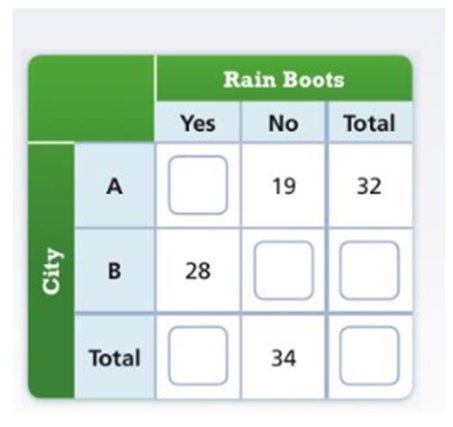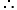Maths-
General
Easy

Question

# A Washerman asks 75 people from two different cities if they own rain boots. Complete thetwo-way frequency table to show the results of the survey.What pattern do you see in the two-way frequency table?Hint:

## The correct answer is: 32,43,75

### Answer:Given: Yes No Total City A a 19 32 City B 28 b d Total c 34 e Step 1: Find a.As, we know,Totals appear at the end of each row and columna + 19 = 32a = 32 – 19a = 13 Step 2: Find c.As, we know,Totals appear at the end of each row and columna + 28 = cc = 28 + 13c = 41 Step 3: Find b.As, we know,Totals appear at the end of each row and columnb + 19 = 34b = 34 – 19b = 15 Step 4: Find dAs, we know,Totals appear at the end of each row and column28 + b = dd = 28 + 15d = 43 Step 5: Find e.As, we know,A sum of all counts (a grand total) is placed at the bottom right32 + d = ee = 32 + 43e = 75 Final Answer: Yes No Total City A 13 19 32 City B 28 15 43 Total 41 34 75#### With Turito Foundation.#### Get an Expert Advice From Turito.